Updated 29/09/2023
In force

Version from: 28/06/2023
Amendments
There is currently no level 2 legal act based on or specifying Article 280f.
Search within this legal act

### Article 280f - Other risks category add-on

Article 280f

1.

For the purposes of Article 278, institutions shall calculate the other risks category add-on for a given netting set as follows: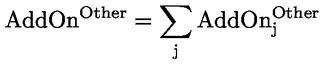where:

 єj = the index that denotes the other risk hedging sets established in accordance with point (f) of Article 277a(1) and Article 277a(2) for the netting set; and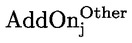= the other risks category add-on for hedging set j calculated in accordance with paragraph 2.
2.

Institutions shall calculate the other risks category add-on for hedging set j as follows: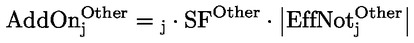where:= the other risks category add-on for hedging set j;
 єj = the hedging set supervisory factor coefficient of hedging set j determined in accordance with Article 280; and
 SFOther = the supervisory factor for the other risk category with a value equal to 8 %;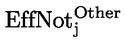=

the effective notional amount of hedging set j calculated as follows: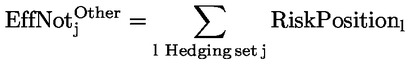where:

 l = the index that denotes the risk position.